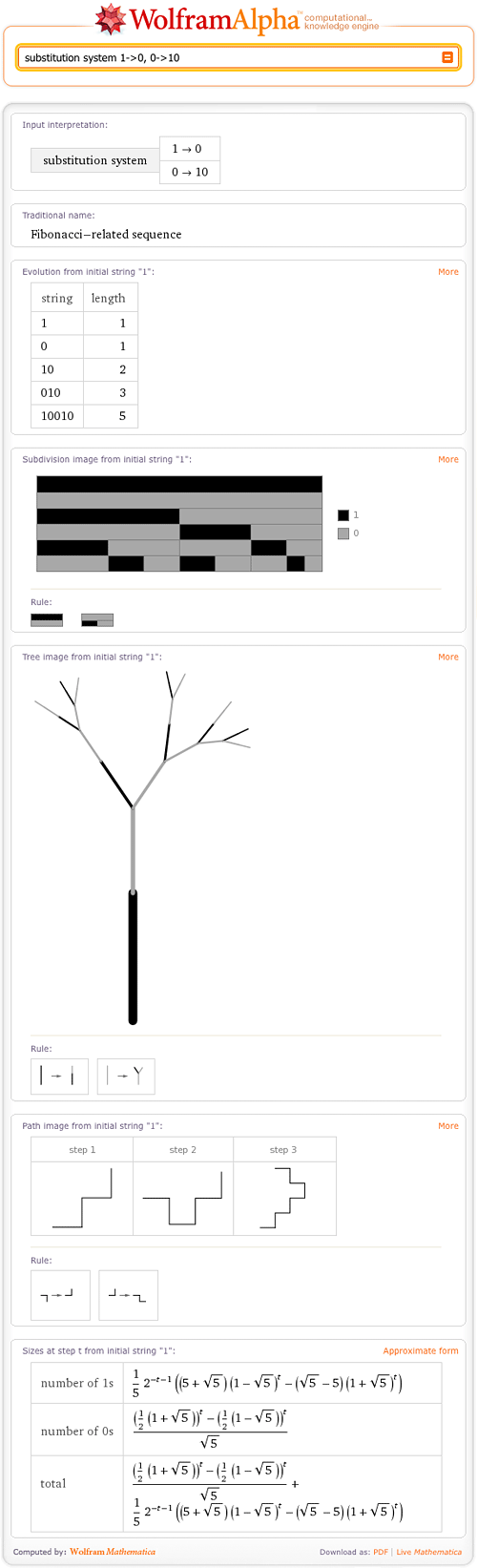TAG: Substitution Systems
February 3, 2010–

When Wolfram|Alpha was introduced, Stephen Wolfram blogged about it being the first “killer app” that resulted from his work on A New Kind of Science (NKS). We can now use this application of NKS to further our exploration and study within the NKS field. For example, one class of systems discussed in NKS is that of substitution systems. Now that a host of string substitution systems have been integrated into Wolfram|Alpha, we can explore a variety of these systems—not just the ones that are well known.

A string substitution system is composed of two parts: a string and a set of rules. The string looks like a series of numbers, say “0” and “1”. The rules describe what happens to each number in the string; for example,  “1” -> “0” and “0” -> “10”. Under our rules, our example string, “1”, transforms to “0”. In true NKS fashion, repeated iteration of these simple rules can give interesting behavior. Our example, which seems deceptively simple, can model the Fibonacci numbers. We simply document the length of the string each time we apply the rules to find that the series of lengths obtained at the end of each substitution corresponds to the Fibonacci series: {1, 1, 2, 3, 5…}. We see this in the following result:Similarly, there is a string substitution system that models the Cantor set. The rules that define this substitution system are 1->101 and 0->000: More »